# R S Aggarwal Solutions for Class 10 Maths Chapter 5 Arithmetic Progression Exercise 5C

R S Aggarwal Solutions for Class 10 Maths exercise 5c are available here. The PDF of the Class 10 Maths Chapter 5 R S Aggarwal Solutions on exercise 5c can be downloaded and practiced online as well as offline. Download pdf of Class 10 Maths Chapter 5 R S Aggarwal Solutions prepared by subject experts.

## Download PDF of R S Aggarwal Solutions for Class 10 Maths Chapter 5 Arithmetic Progression Exercise 5C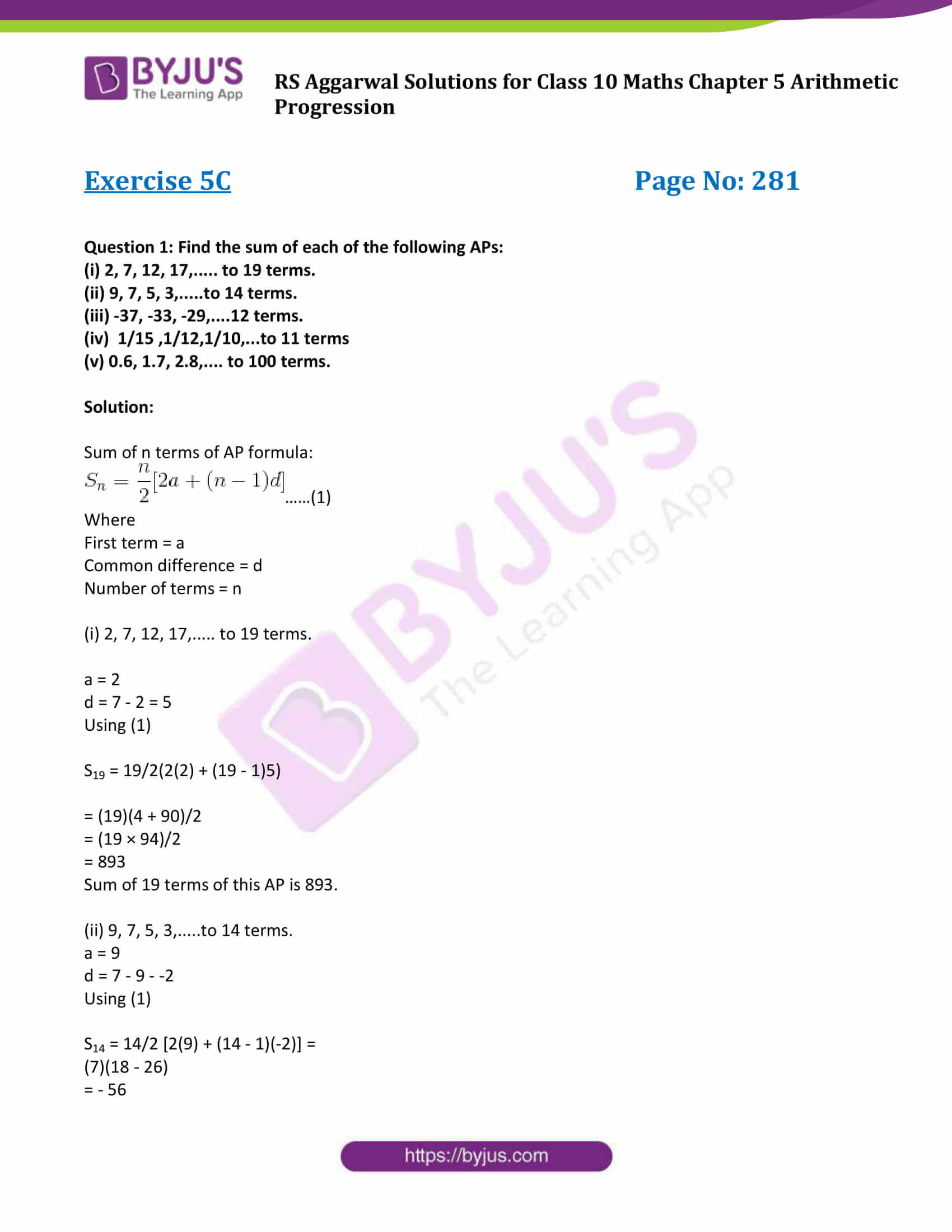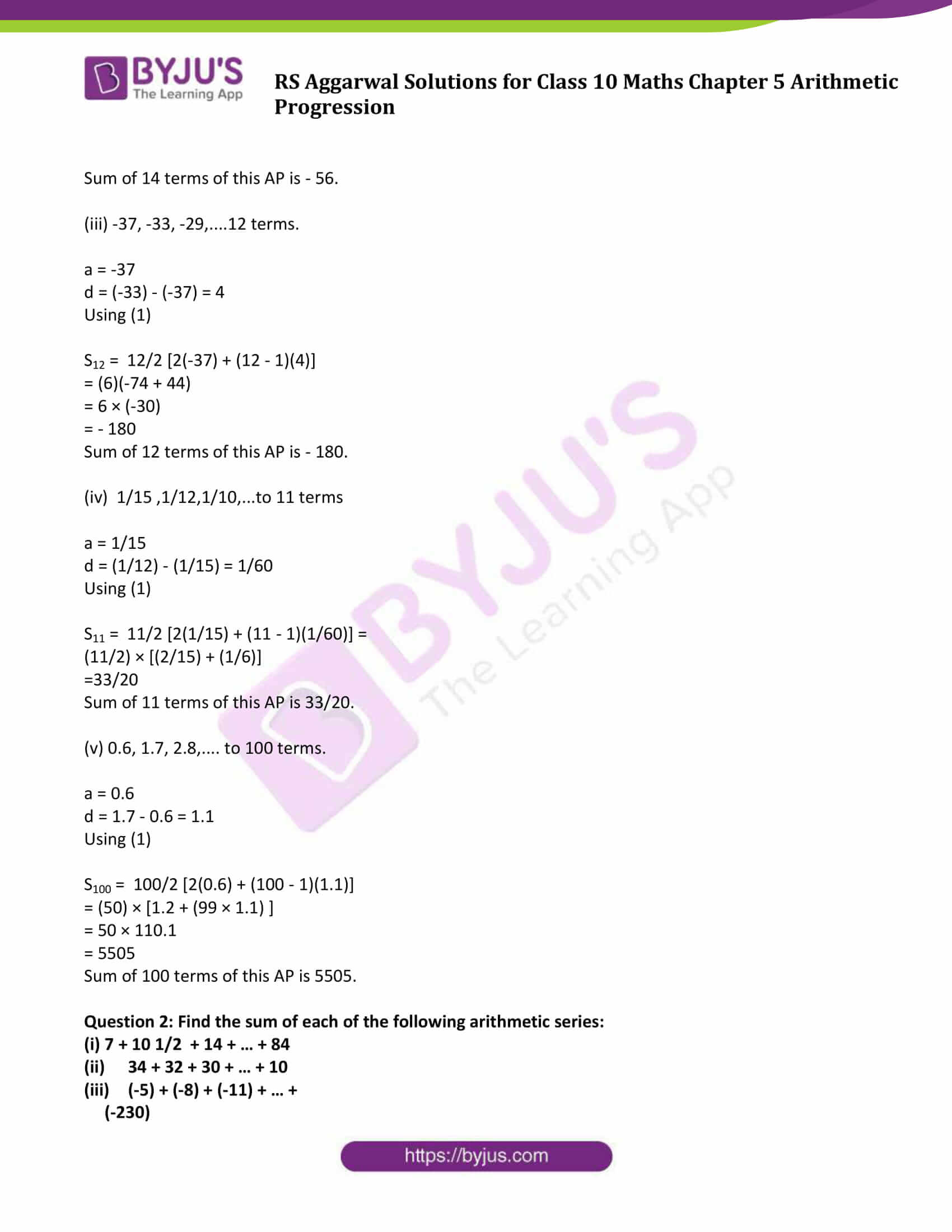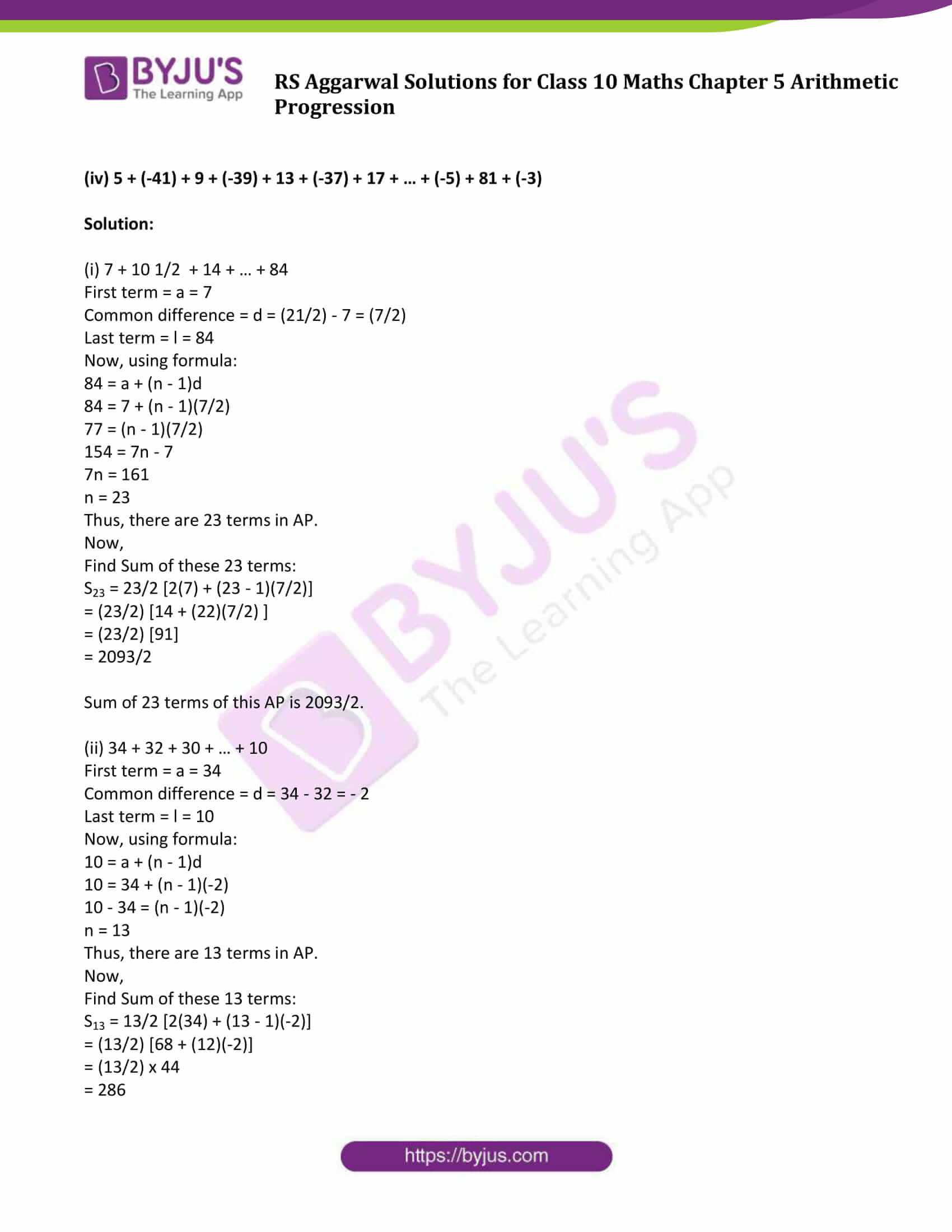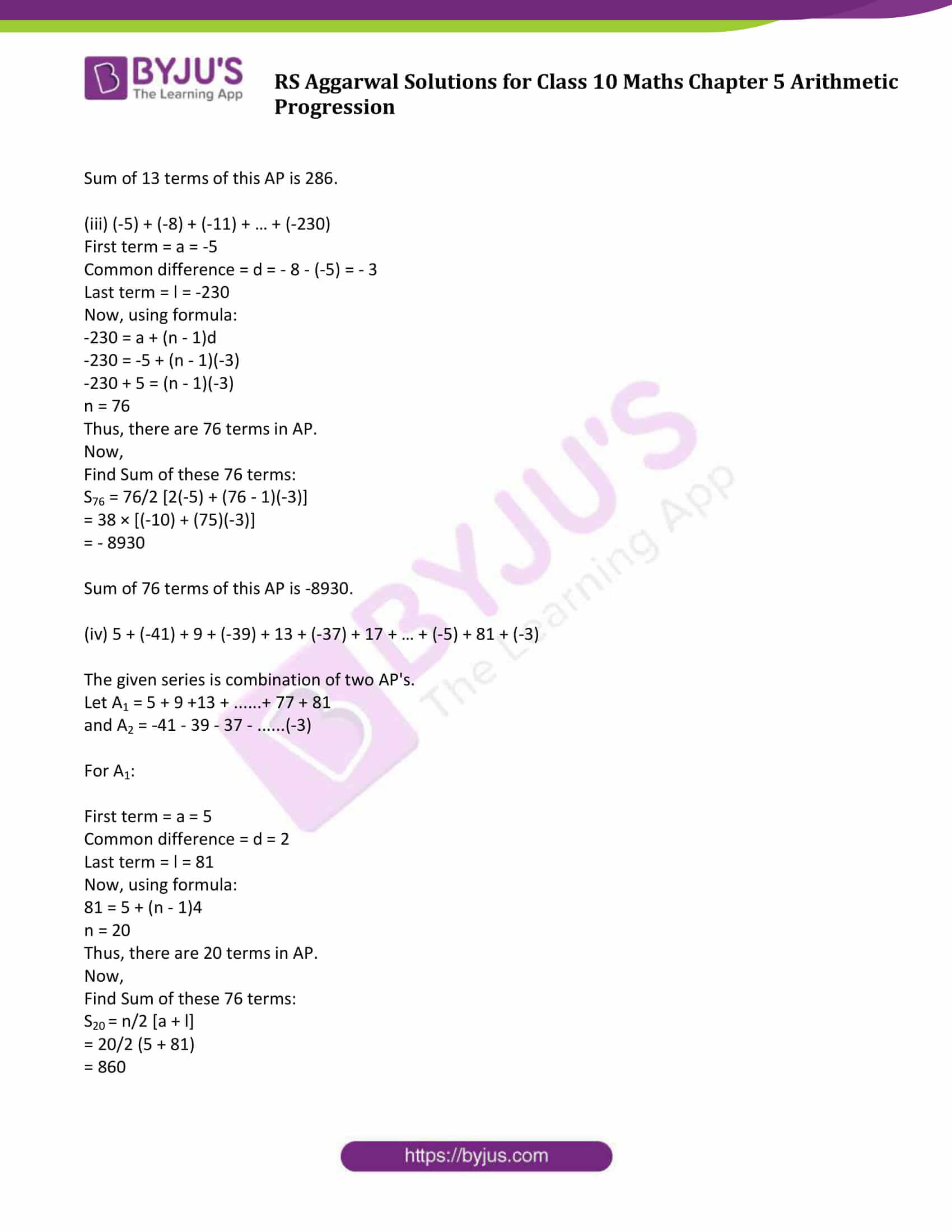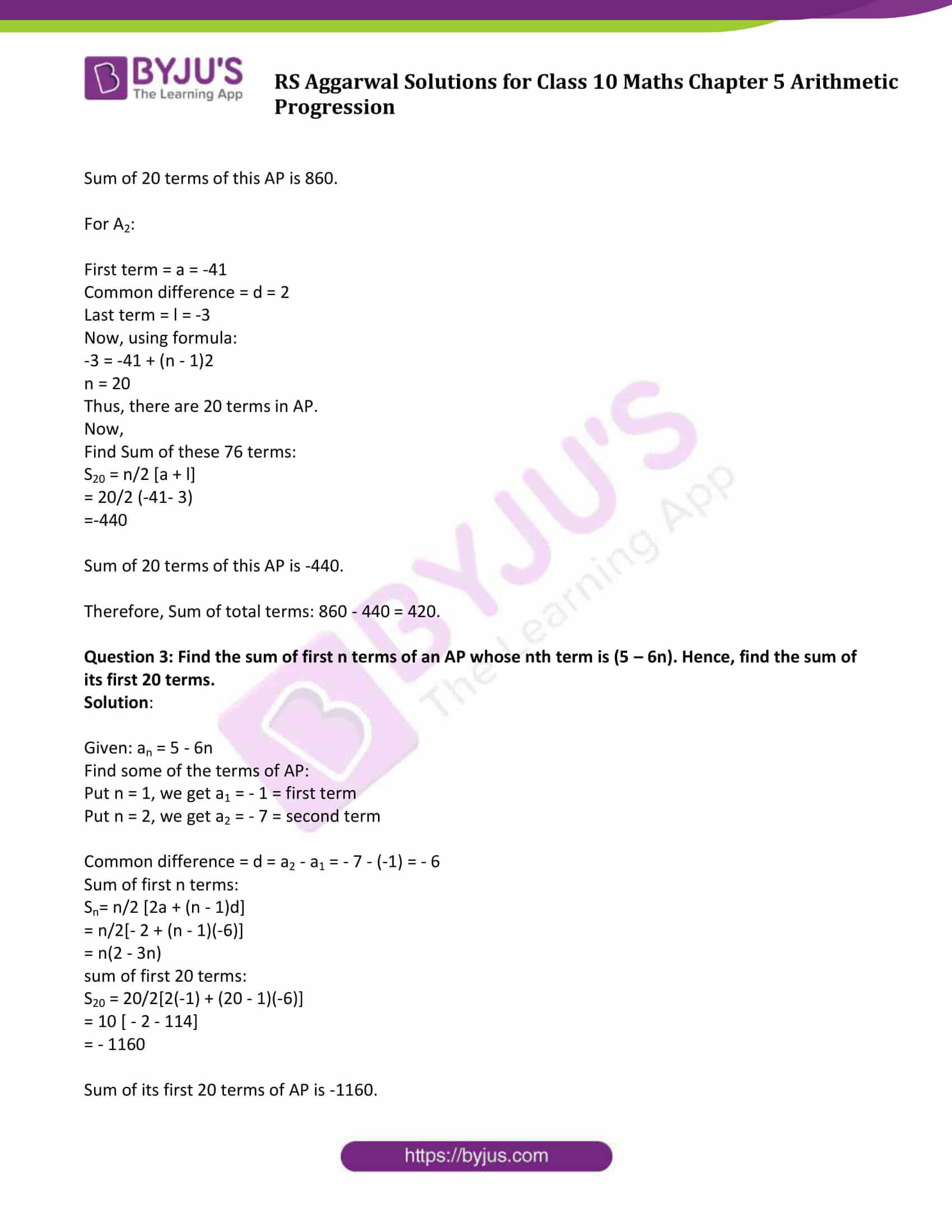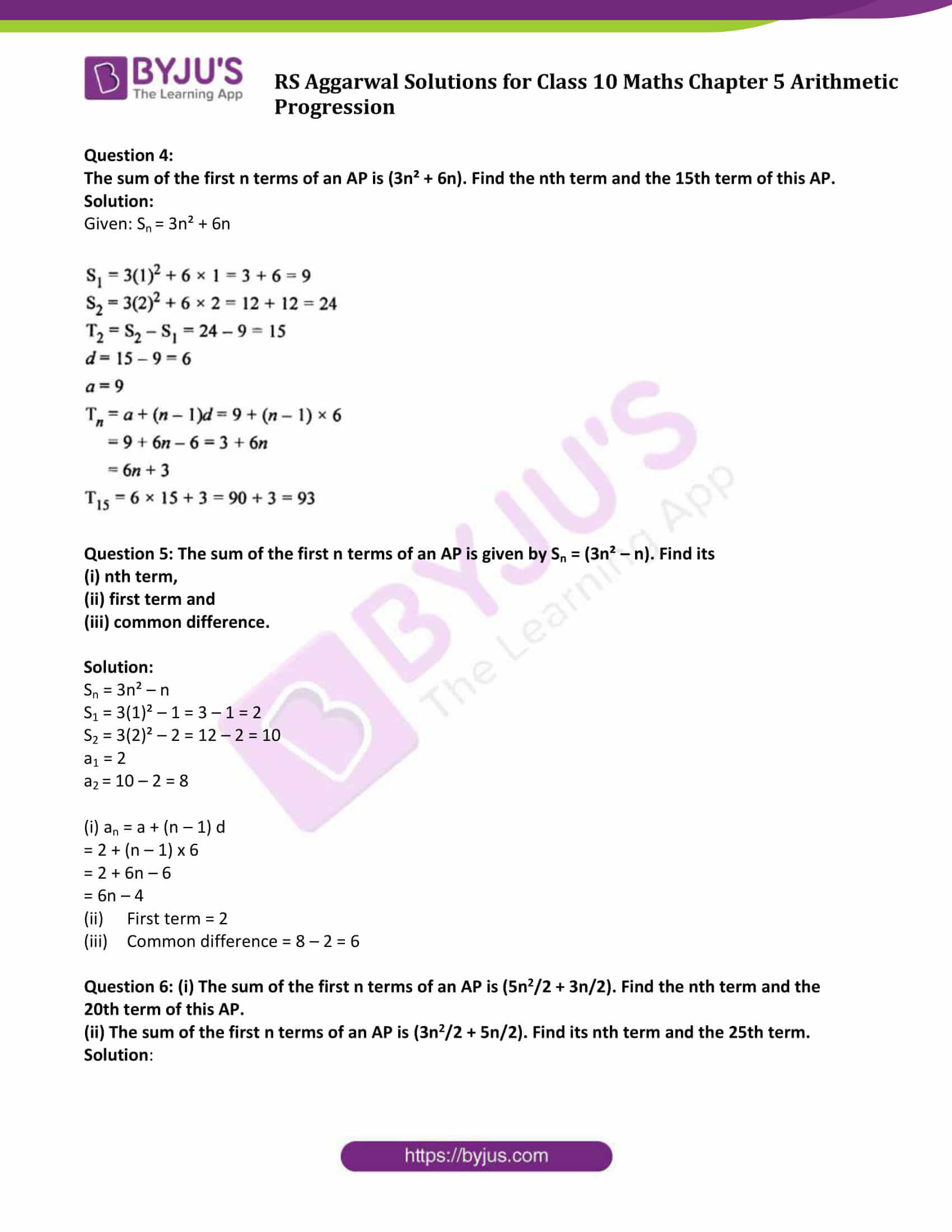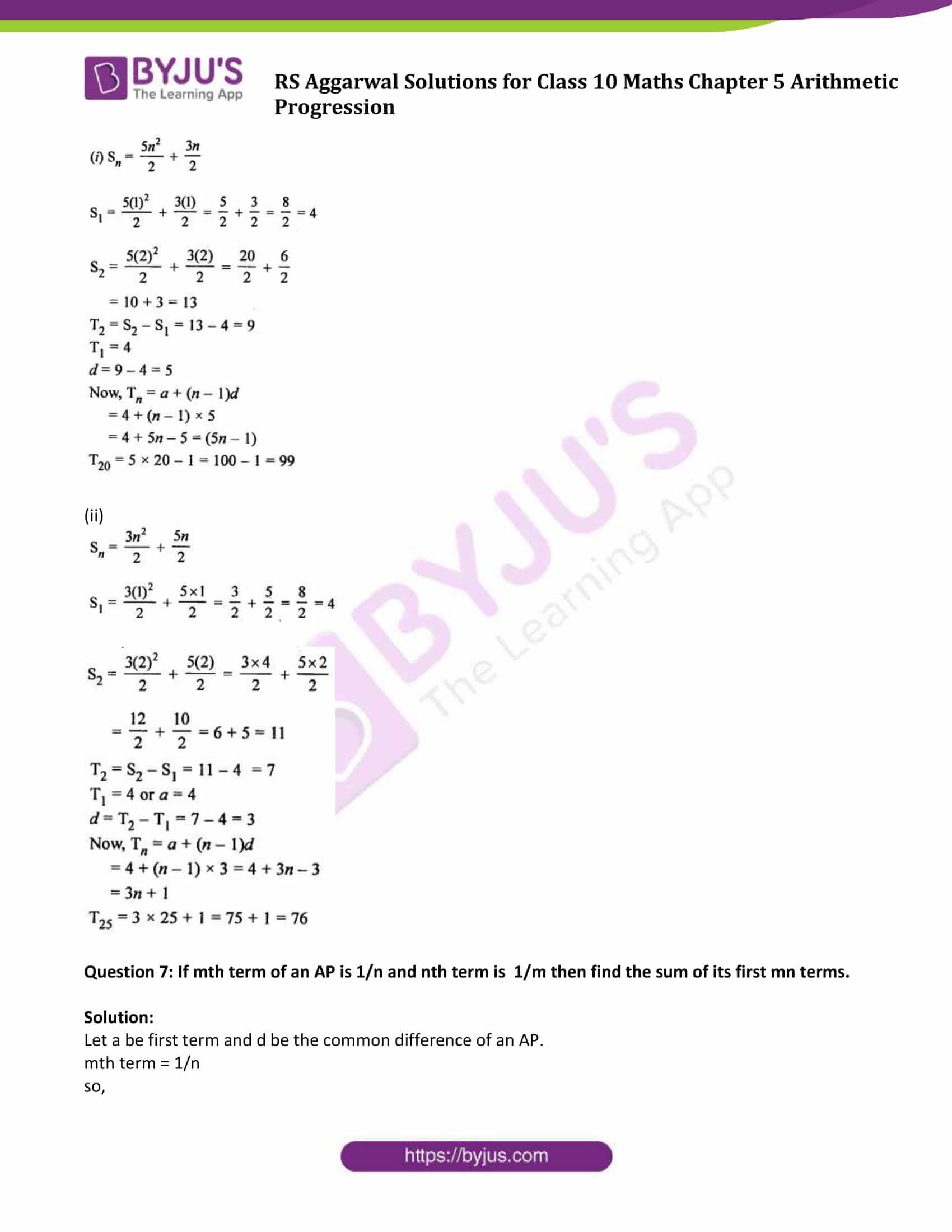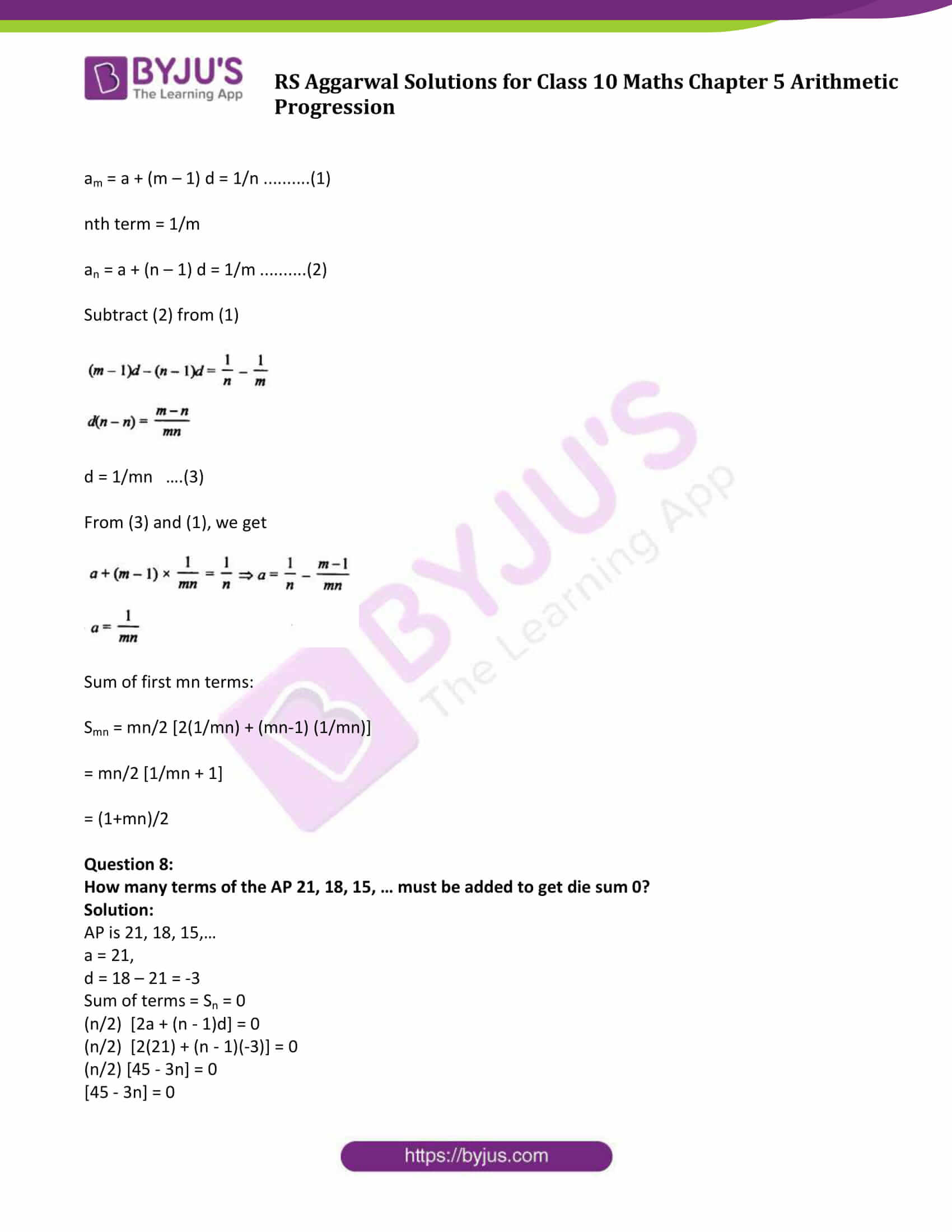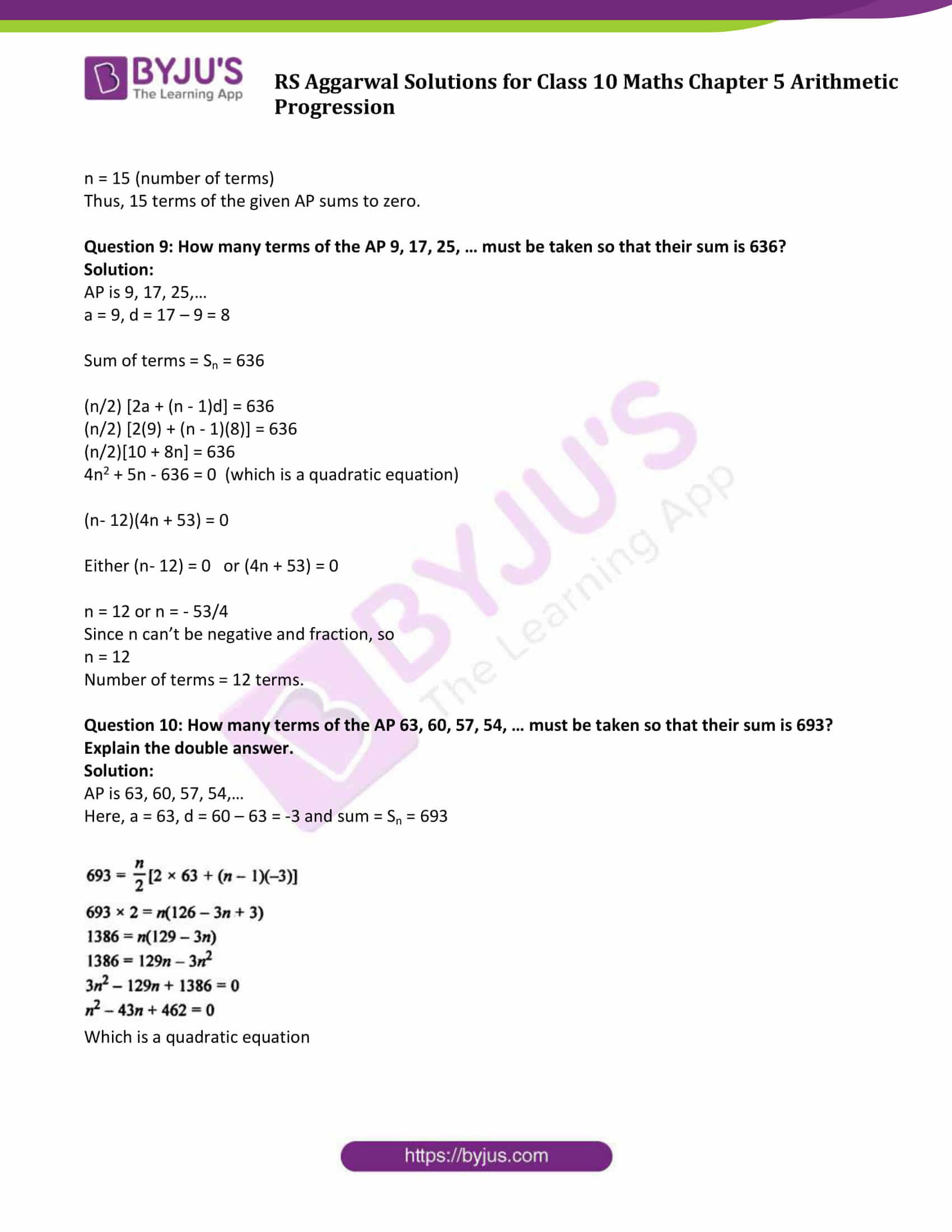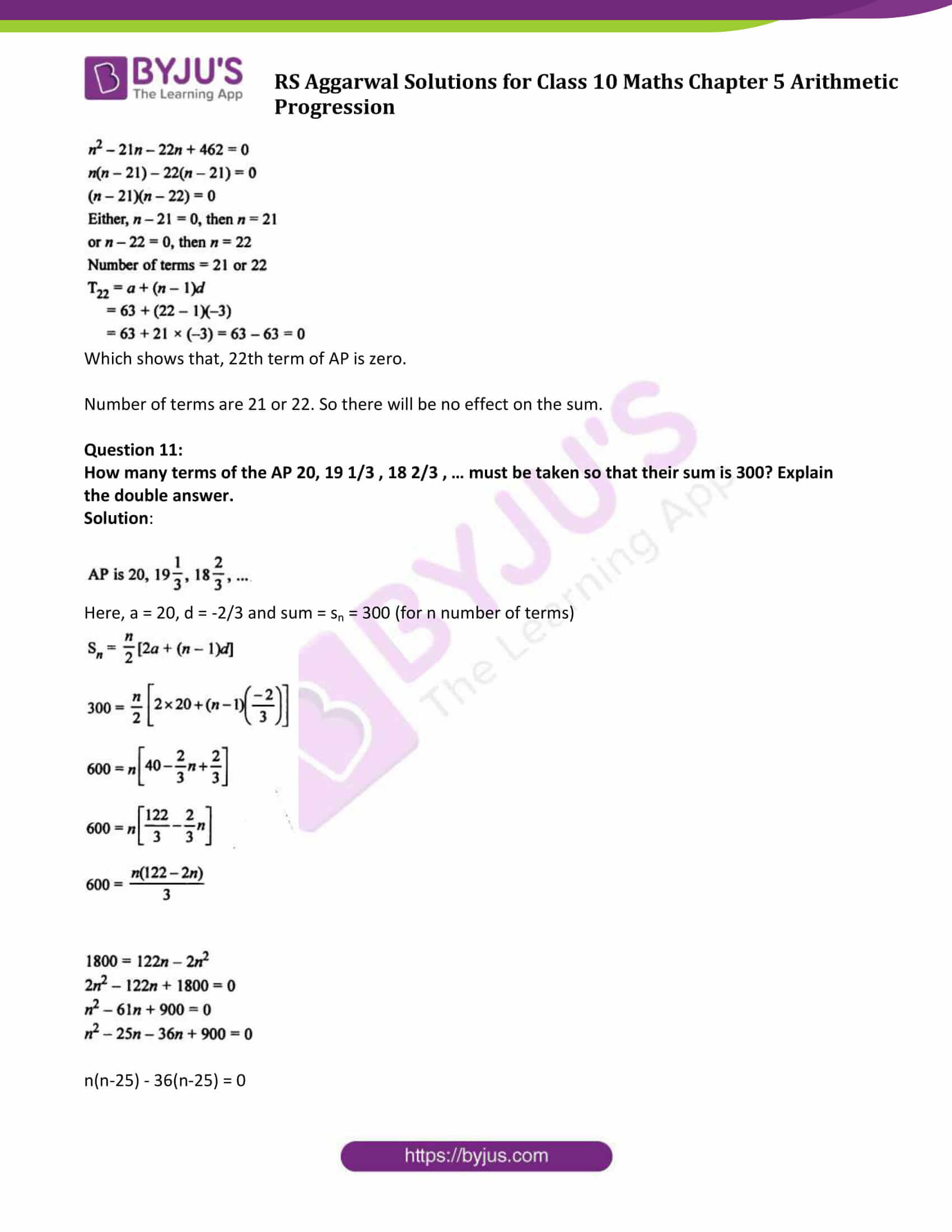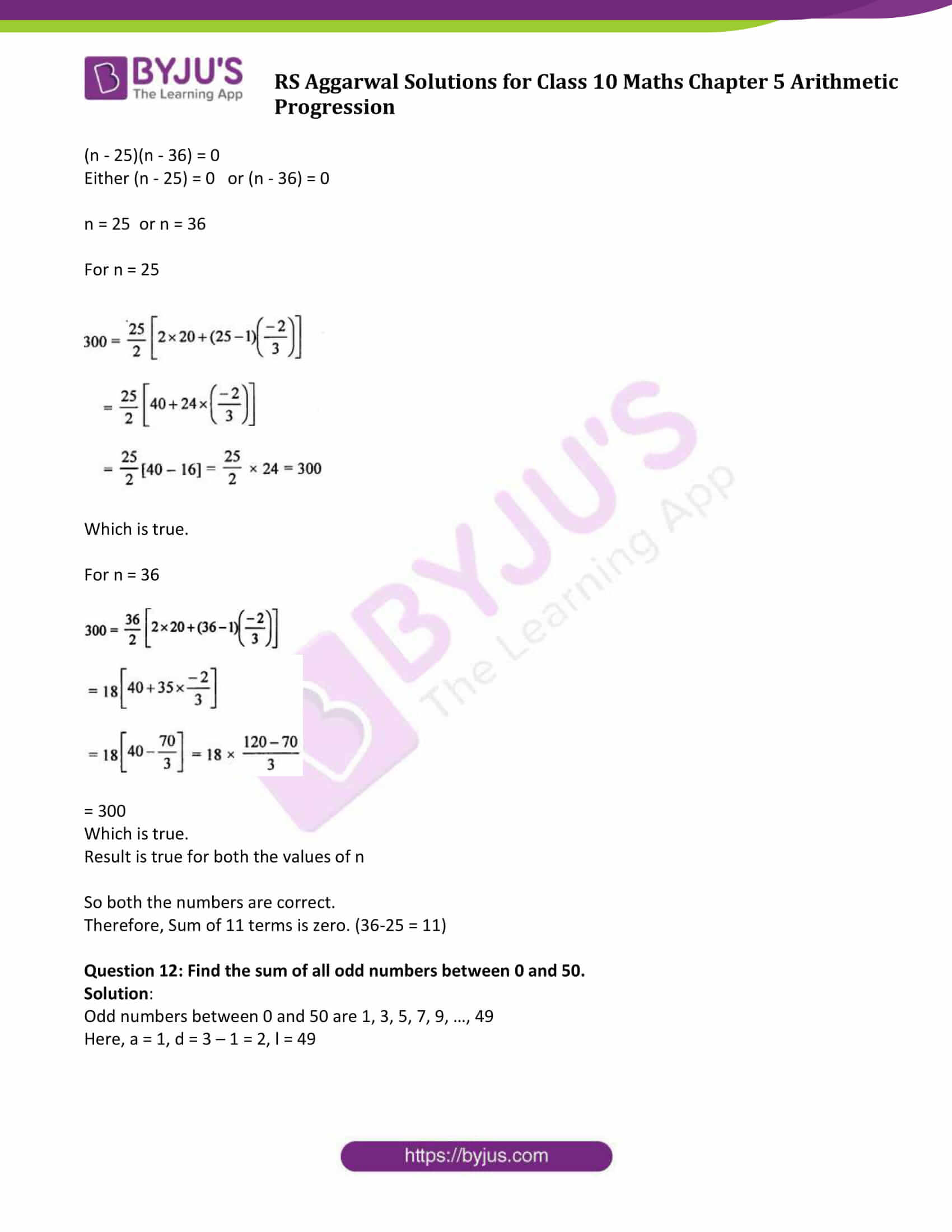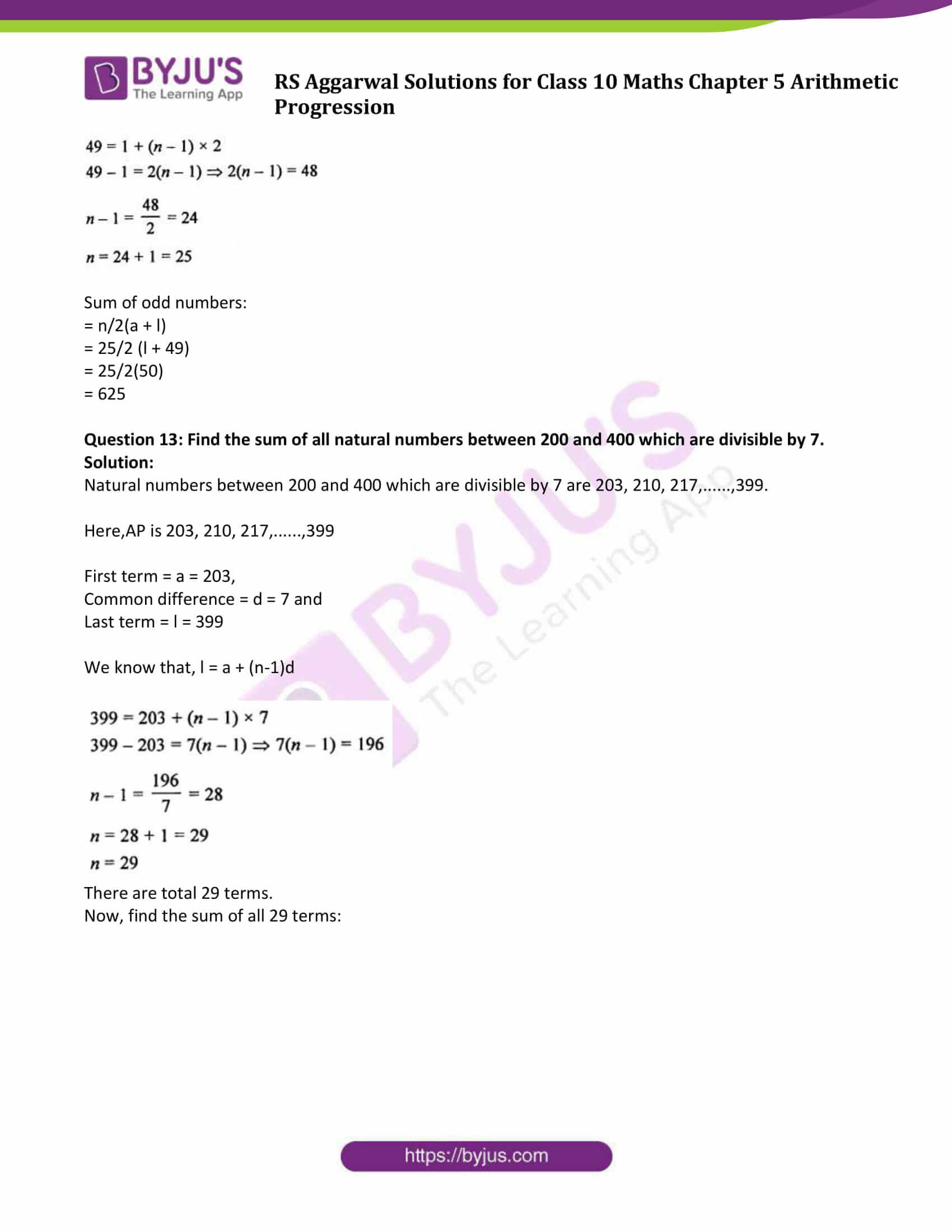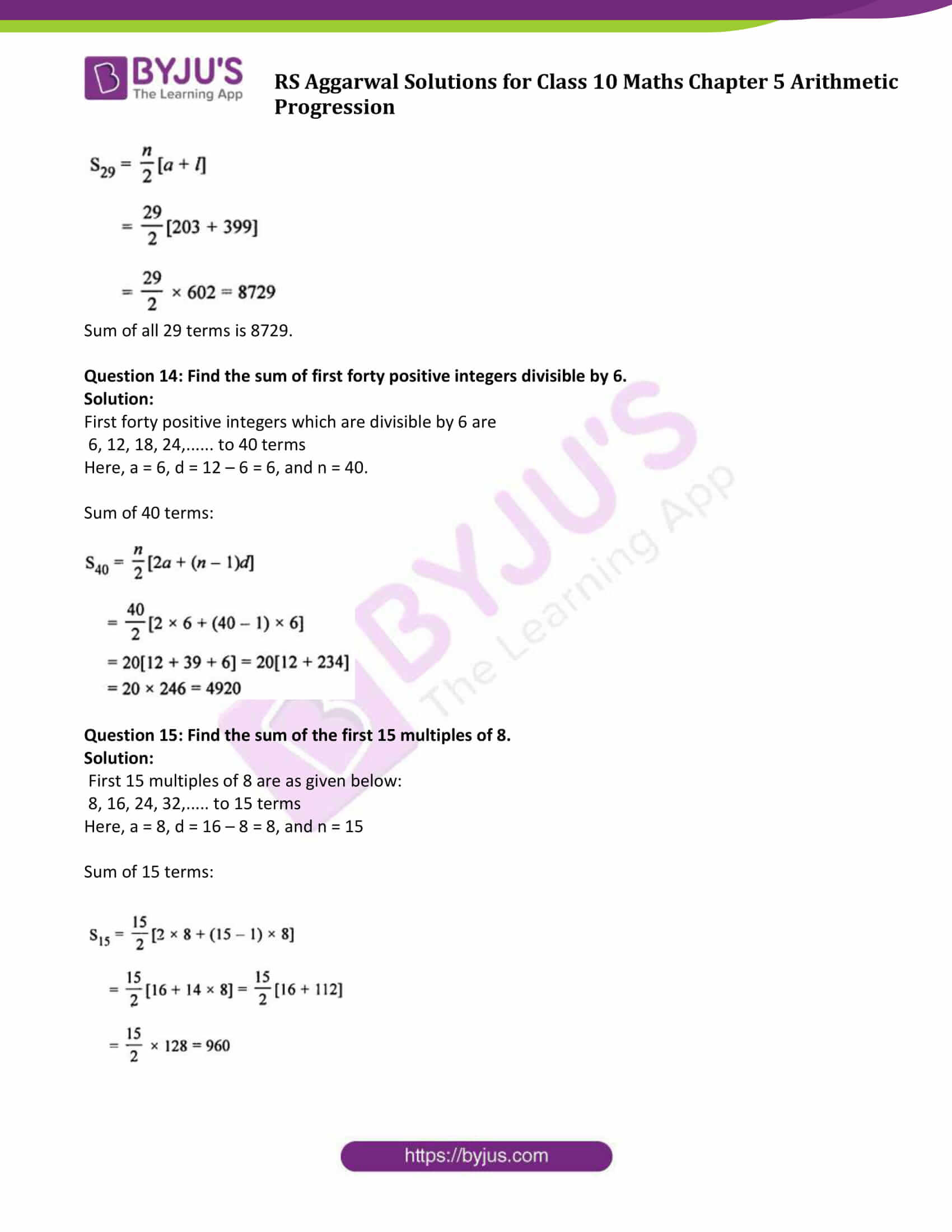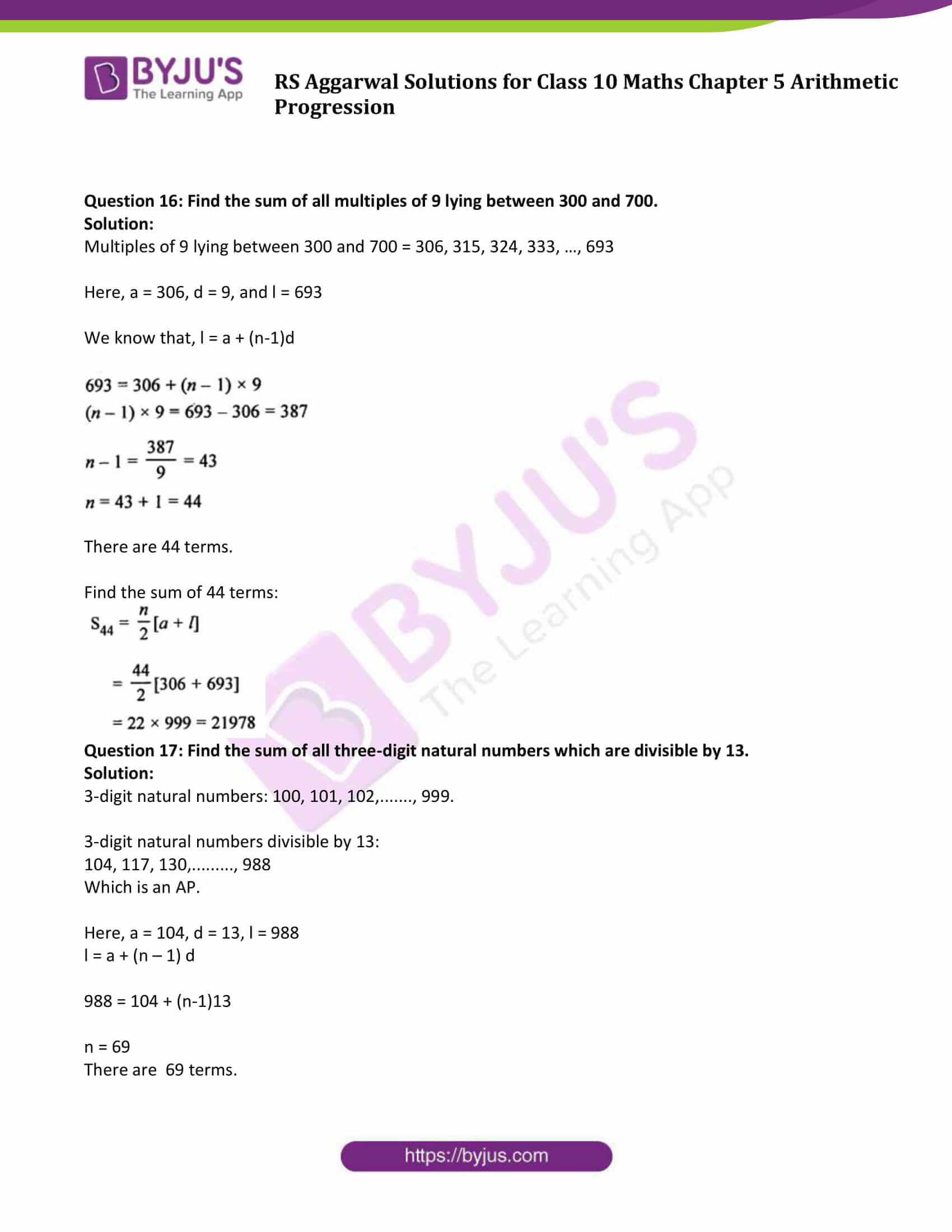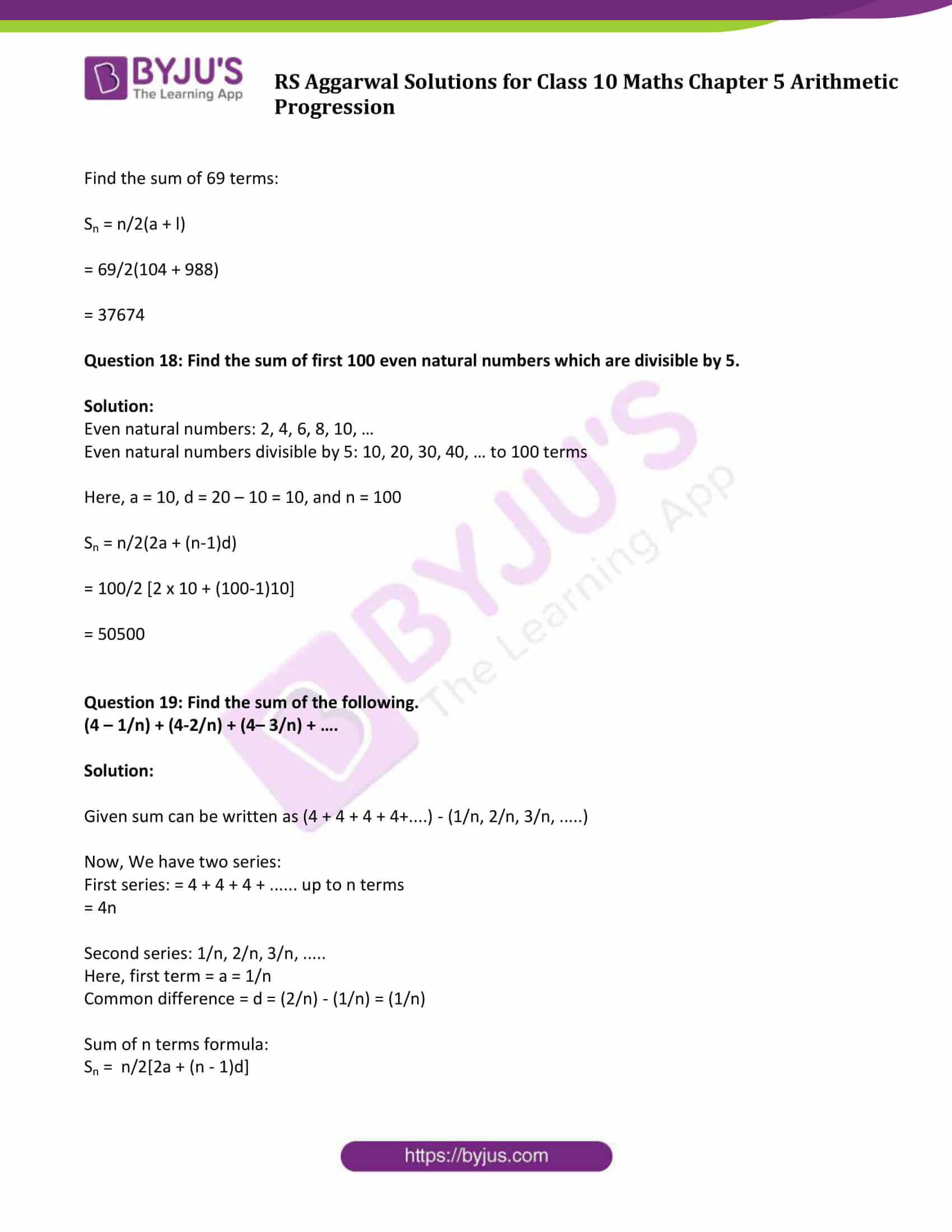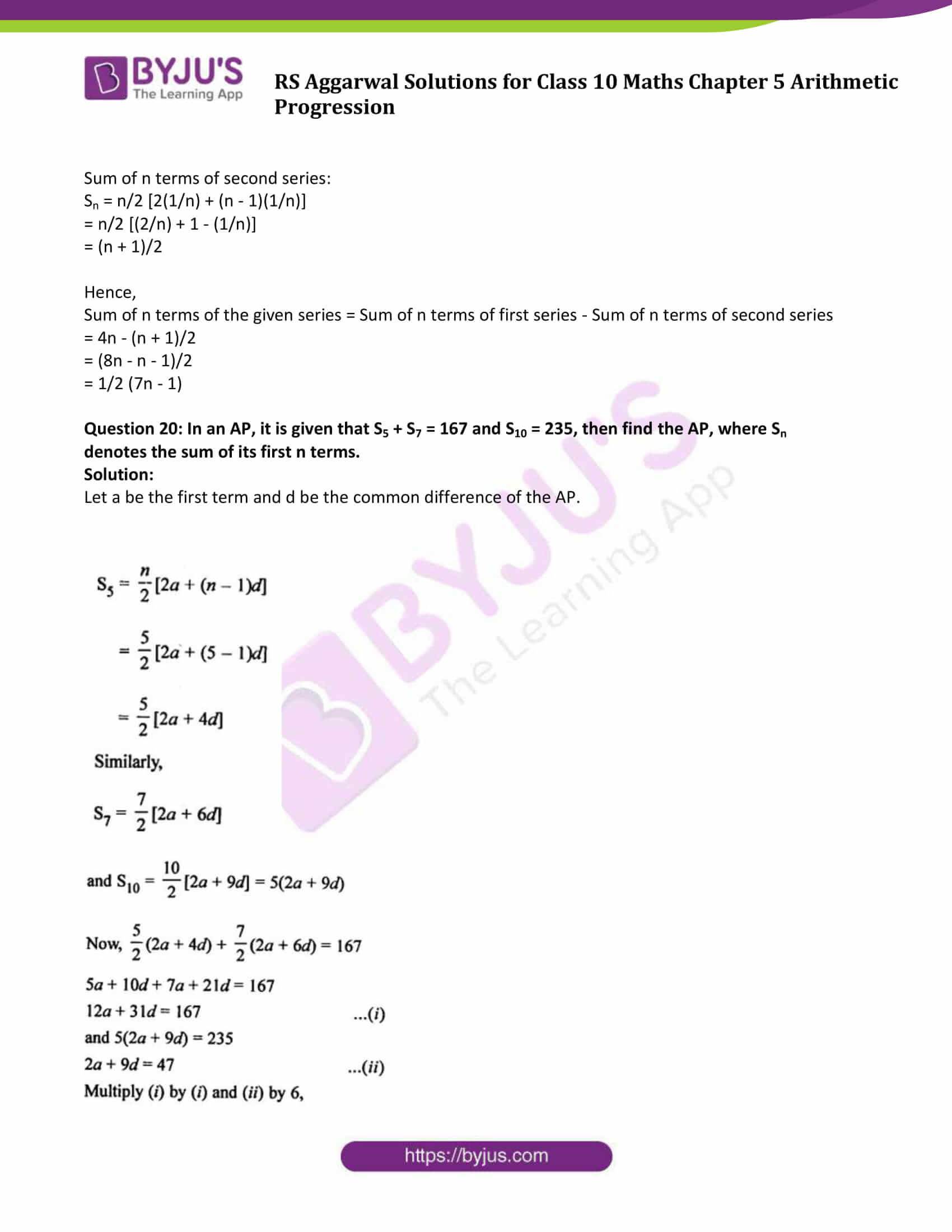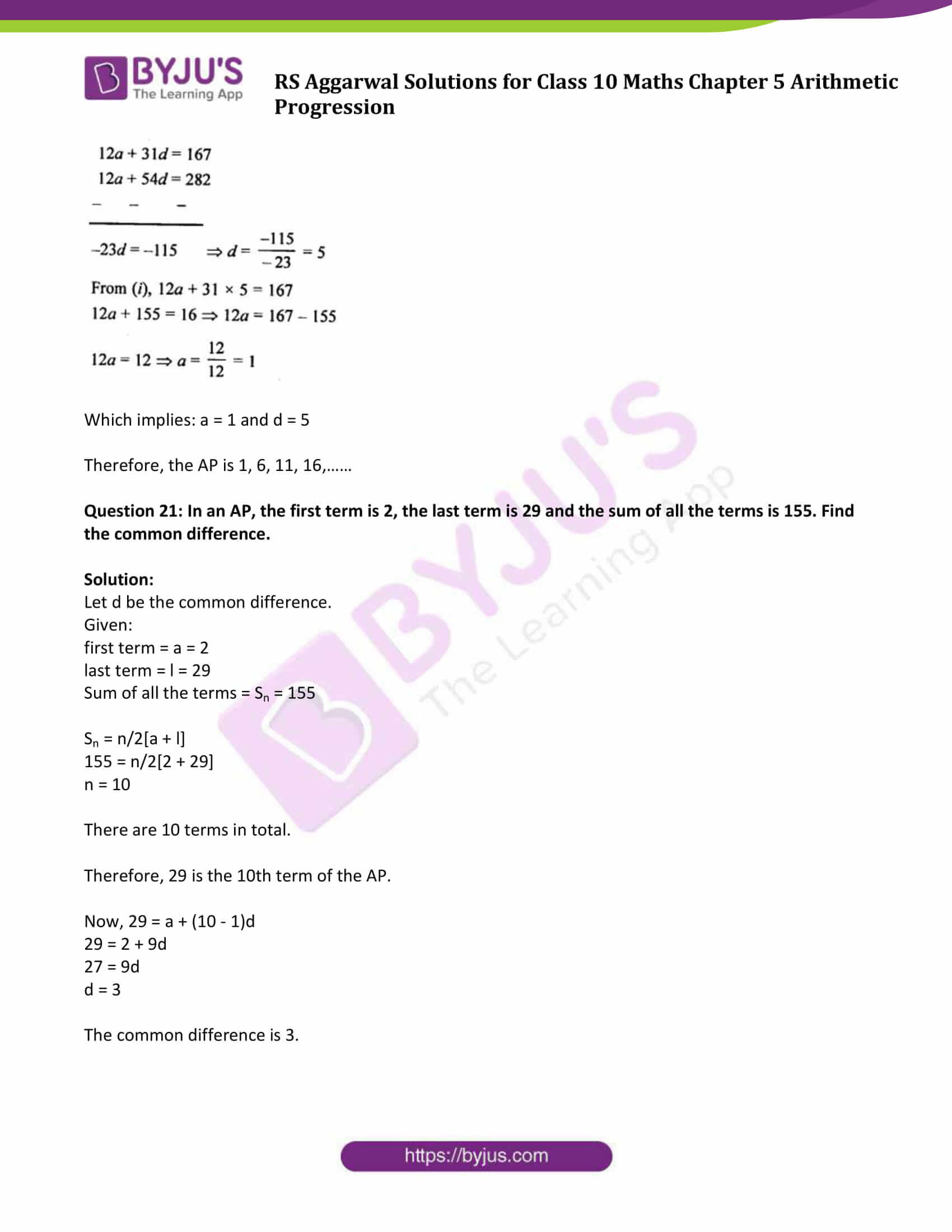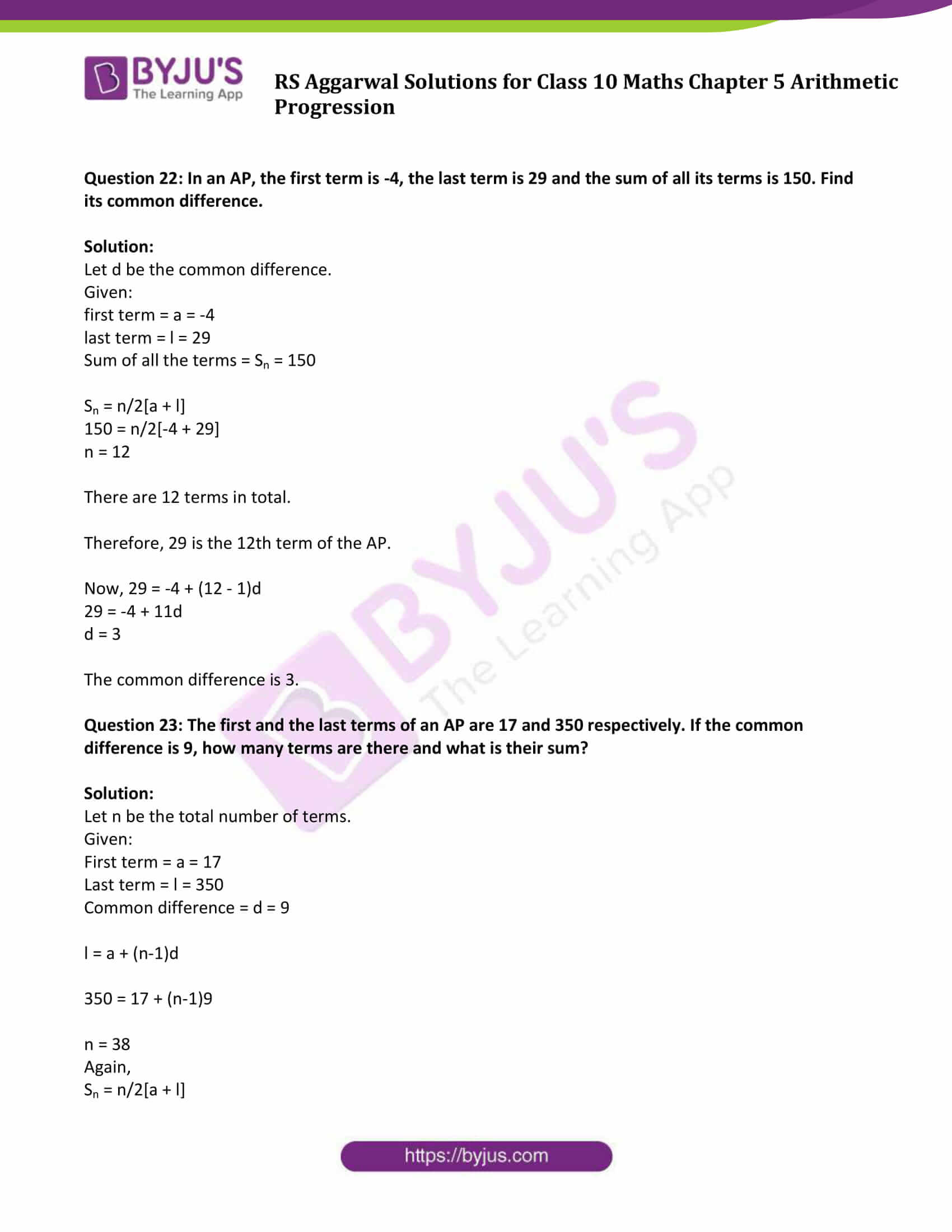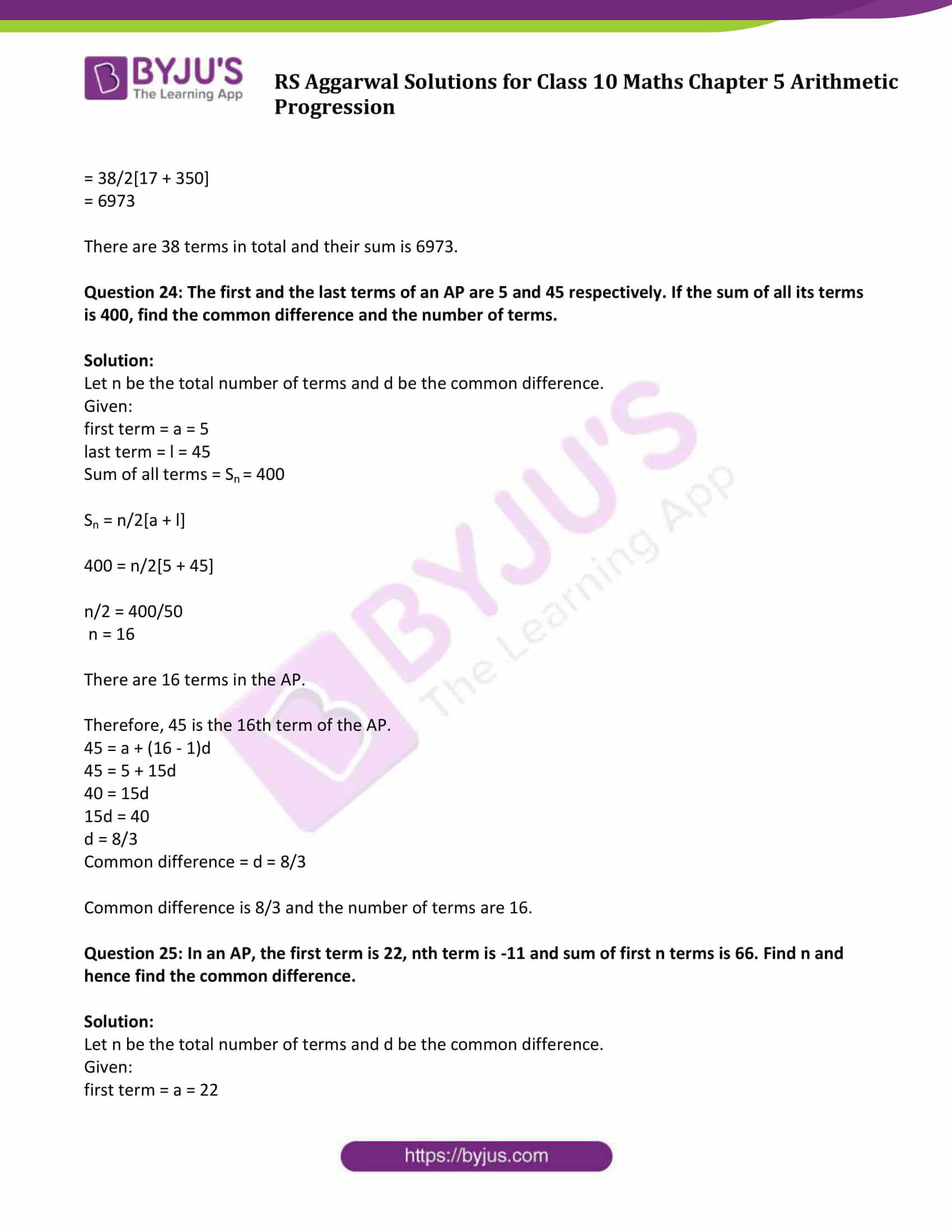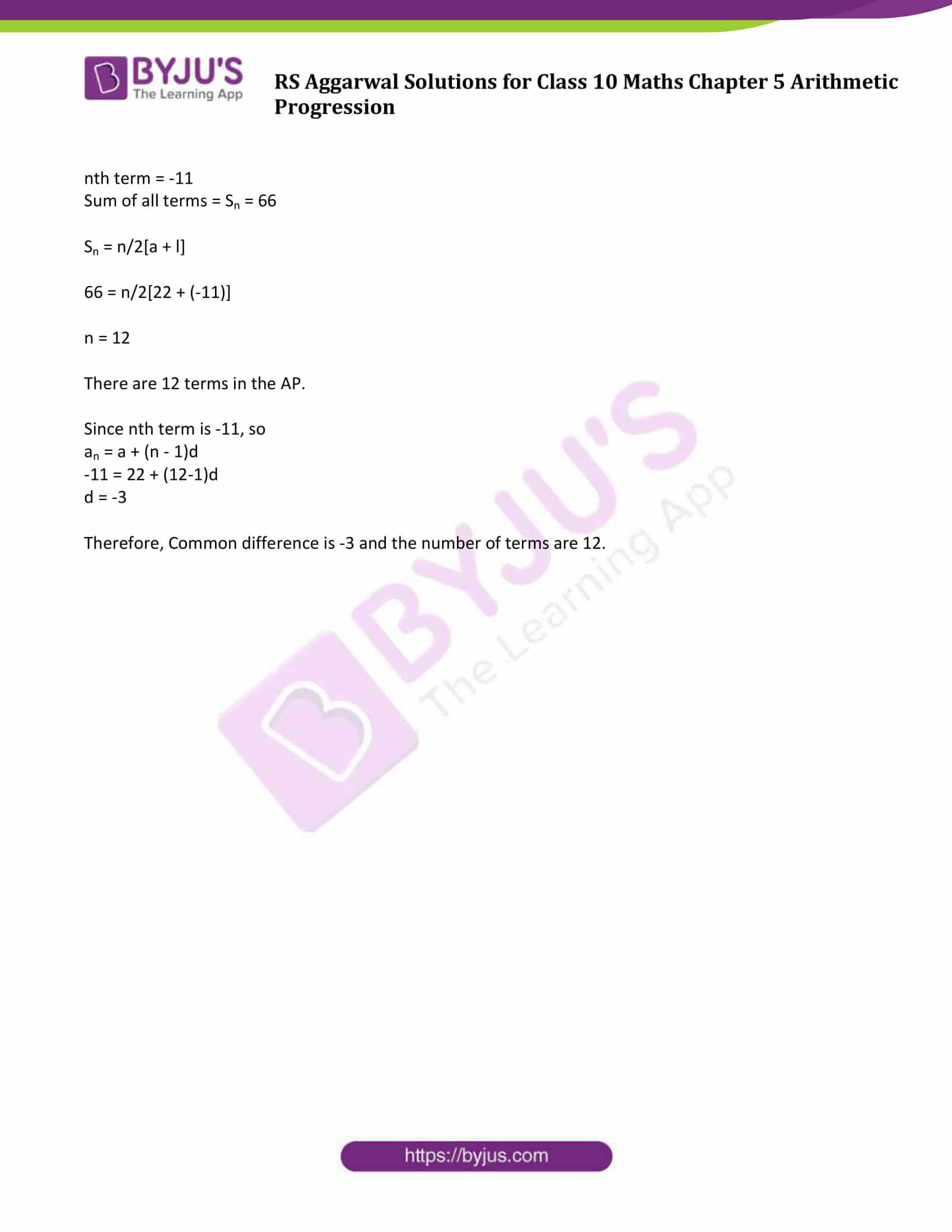### Access other exercise solutions of Class 10 Maths Chapter 5 Arithmetic Progression

Exercise 5A Solutions: 30 Questions (Short Answers)

Exercise 5B Solutions: 16 Questions (Short Answers)

Exercise 5D Solutions: 20 Questions (Long Answers)

## Exercise 5C Page No: 281

Question 1: Find the sum of each of the following APs:

(i) 2, 7, 12, 17,….. to 19 terms.

(ii) 9, 7, 5, 3,…..to 14 terms.

(iii) -37, -33, -29,….12 terms.

(iv) 1/15 ,1/12,1/10,…to 11 terms

(v) 0.6, 1.7, 2.8,…. to 100 terms.

Solution:

Sum of n terms of AP formula: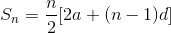……(1)

Where

First term = a

Common difference = d

Number of terms = n

(i) 2, 7, 12, 17,….. to 19 terms.

a = 2

d = 7 – 2 = 5

Using (1)

S19 = 19/2(2(2) + (19 – 1)5)

= (19)(4 + 90)/2

= (19 × 94)/2

= 893

Sum of 19 terms of this AP is 893.

(ii) 9, 7, 5, 3,…..to 14 terms.

a = 9

d = 7 – 9 = -2

Using (1)

S14 = 14/2 [2(9) + (14 – 1)(-2)]

= (7)(18 – 26)

= – 56

Sum of 14 terms of this AP is – 56.

(iii) -37, -33, -29,….12 terms.

a = -37

d = (-33) – (-37) = 4

Using (1)

S12 = 12/2 [2(-37) + (12 – 1)(4)]

= (6)(-74 + 44)

= 6 × (-30)

= – 180

Sum of 12 terms of this AP is – 180.

(iv) 1/15 ,1/12,1/10,…to 11 terms

a = 1/15

d = (1/12) – (1/15) = 1/60

Using (1)

S11 = 11/2 [2(1/15) + (11 – 1)(1/60)]

= (11/2) × [(2/15) + (1/6)]

=33/20

Sum of 11 terms of this AP is 33/20.

(v) 0.6, 1.7, 2.8,…. to 100 terms.

a = 0.6

d = 1.7 – 0.6 = 1.1

Using (1)

S100 = 100/2 [2(0.6) + (100 – 1)(1.1)]

= (50) × [1.2 + (99 × 1.1) ]

= 50 × 110.1

= 5505

Sum of 100 terms of this AP is 5505.

Question 2: Find the sum of each of the following arithmetic series:

(i) 7 + 10 1/2 + 14 + … + 84

(ii) 34 + 32 + 30 + … + 10

(iii) (-5) + (-8) + (-11) + … + (-230)

(iv) 5 + (-41) + 9 + (-39) + 13 + (-37) + 17 + … + (-5) + 81 + (-3)

Solution:

(i) 7 + 10 1/2 + 14 + … + 84

First term = a = 7

Common difference = d = (21/2) – 7 = (7/2)

Last term = l = 84

Now, using formula:

84 = a + (n – 1)d

84 = 7 + (n – 1)(7/2)

77 = (n – 1)(7/2)

154 = 7n – 7

7n = 161

n = 23

Thus, there are 23 terms in AP.

Now,

Find Sum of these 23 terms:

S23 = 23/2 [2(7) + (23 – 1)(7/2)]

= (23/2) [14 + (22)(7/2) ]

= (23/2) 

= 2093/2

Sum of 23 terms of this AP is 2093/2.

(ii) 34 + 32 + 30 + … + 10

First term = a = 34

Common difference = d = 34 – 32 = – 2

Last term = l = 10

Now, using formula:

10 = a + (n – 1)d

10 = 34 + (n – 1)(-2)

10 – 34 = (n – 1)(-2)

n = 13

Thus, there are 13 terms in AP.

Now,

Find Sum of these 13 terms:

S13 = 13/2 [2(34) + (13 – 1)(-2)]

= (13/2) [68 + (12)(-2)]

= (13/2) x 44

= 286

Sum of 13 terms of this AP is 286.

(iii) (-5) + (-8) + (-11) + … + (-230)

First term = a = -5

Common difference = d = – 8 – (-5) = – 3

Last term = l = -230

Now, using formula:

-230 = a + (n – 1)d

-230 = -5 + (n – 1)(-3)

– 230 + 5 = (n – 1)(-3)

n = 76

Thus, there are 76 terms in AP.

Now,

Find Sum of these 76 terms:

S76 = 76/2 [2(-5) + (76 – 1)(-3)]

= 38 × [(-10) + (75)(-3)]

= – 8930

Sum of 76 terms of this AP is -8930.

(iv) 5 + (-41) + 9 + (-39) + 13 + (-37) + 17 + … + (-5) + 81 + (-3)

The given series is combination of two AP’s.

Let A1 = 5 + 9 +13 + ……+ 77 + 81

and A2 = -41 – 39 – 37 – ……(-3)

For A1:

First term = a = 5

Common difference = d = 2

Last term = l = 81

Now, using formula:

81 = 5 + (n – 1)4

n = 20

Thus, there are 20 terms in AP.

Now,

Find Sum of these 76 terms:

S20 = n/2 [a + l]

= 20/2 (5 + 81)

= 860

Sum of 20 terms of this AP is 860.

For A2:

First term = a = -41

Common difference = d = 2

Last term = l = -3

Now, using formula:

-3 = -41 + (n – 1)2

n = 20

Thus, there are 20 terms in AP.

Now,

Find Sum of these 76 terms:

S20 = n/2 [a + l]

= 20/2 (-41- 3)

=-440

Sum of 20 terms of this AP is -440.

Therefore, Sum of total terms: 860 – 440 = 420.

Question 3: Find the sum of first n terms of an AP whose nth term is (5 – 6n). Hence, find the sum of its first 20 terms.

Solution:

Given: an = 5 – 6n

Find some of the terms of AP:

Put n = 1, we get a1 = – 1 = first term

Put n = 2, we get a2 = – 7 = second term

Common difference = d = a2 – a1 = – 7 – (-1) = – 6

Sum of first n terms:

Sn= n/2 [2a + (n – 1)d]

= n/2[- 2 + (n – 1)(-6)]

= n(2 – 3n)

sum of first 20 terms:

S20 = 20/2[2(-1) + (20 – 1)(-6)]

= 10 [ – 2 – 114]

= – 1160

Sum of its first 20 terms of AP is -1160.

Question 4:

The sum of the first n terms of an AP is (3n² + 6n). Find the nth term and the 15th term of this AP.

Solution:

Given: Sn = 3n² + 6n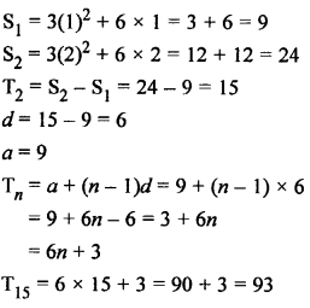Question 5: The sum of the first n terms of an AP is given by Sn = (3n² – n). Find its

(i) nth term,

(ii) first term and

(iii) common difference.

Solution:

Sn = 3n² – n

S1 = 3(1)² – 1 = 3 – 1 = 2

S2 = 3(2)² – 2 = 12 – 2 = 10

a1 = 2

a2 = 10 – 2 = 8

(i) an = a + (n – 1) d

= 2 + (n – 1) x 6

= 2 + 6n – 6

= 6n – 4

(ii) First term = 2

(iii) Common difference = 8 – 2 = 6

Question 6: (i) The sum of the first n terms of an AP is (5n2/2 + 3n/2). Find the nth term and the 20th term of this AP.

(ii) The sum of the first n terms of an AP is (3n2/2 + 5n/2). Find its nth term and the 25th term.

Solution: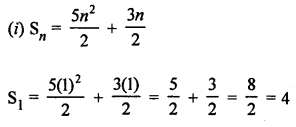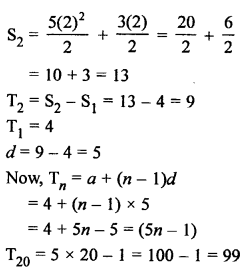(ii)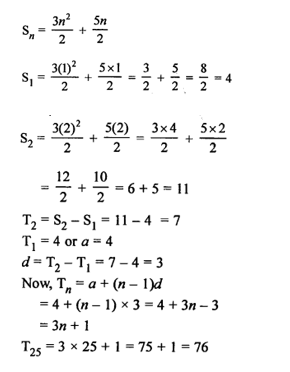Question 7: If mth term of an AP is 1/n and nth term is 1/m then find the sum of its first mn terms.

Solution:

Let a be first term and d be the common difference of an AP.

mth term = 1/n

so,

am = a + (m – 1) d = 1/n ……….(1)

nth term = 1/m

an = a + (n – 1) d = 1/m ……….(2)

Subtract (2) from (1)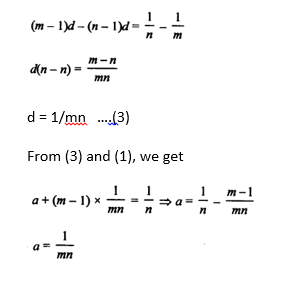Sum of first mn terms:

Smn = mn/2 [2(1/mn) + (mn-1) (1/mn)]

= mn/2 [1/mn + 1]

= (1+mn)/2

Question 8:

How many terms of the AP 21, 18, 15, … must be added to get die sum 0?

Solution:

AP is 21, 18, 15,…

a = 21,

d = 18 – 21 = -3

Sum of terms = Sn = 0

(n/2) [2a + (n – 1)d] = 0

(n/2) [2(21) + (n – 1)(-3)] = 0

(n/2) [45 – 3n] = 0

[45 – 3n] = 0

n = 15 (number of terms)

Thus, 15 terms of the given AP sums to zero.

Question 9: How many terms of the AP 9, 17, 25, … must be taken so that their sum is 636?

Solution:

AP is 9, 17, 25,…

a = 9, d = 17 – 9 = 8

Sum of terms = Sn = 636

(n/2) [2a + (n – 1)d] = 636

(n/2) [2(9) + (n – 1)(8)] = 636

(n/2)[10 + 8n] = 636

4n2 + 5n – 636 = 0 (which is a quadratic equation)

(n- 12)(4n + 53) = 0

Either (n- 12) = 0 or (4n + 53) = 0

n = 12 or n = – 53/4

Since n can’t be negative and fraction, so

n = 12

Number of terms = 12 terms.

Question 10: How many terms of the AP 63, 60, 57, 54, … must be taken so that their sum is 693? Explain the double answer.

Solution:

AP is 63, 60, 57, 54,…

Here, a = 63, d = 60 – 63 = -3 and sum = Sn = 693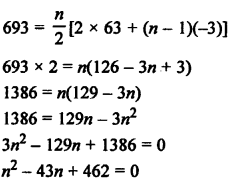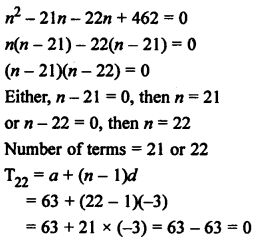Which shows that, 22th term of AP is zero.

Number of terms are 21 or 22. So there will be no effect on the sum.

Question 11:

How many terms of the AP 20, 19 1/3 , 18 2/3 , … must be taken so that their sum is 300? Explain the double answer.

Solution:Here, a = 20, d = -2/3 and sum = sn = 300 (for n number of terms)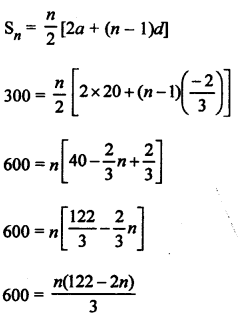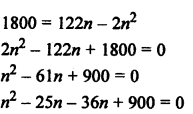n(n-25) – 36(n-25) = 0

(n – 25)(n – 36) = 0

Either (n – 25) = 0 or (n – 36) = 0

n = 25 or n = 36

For n = 25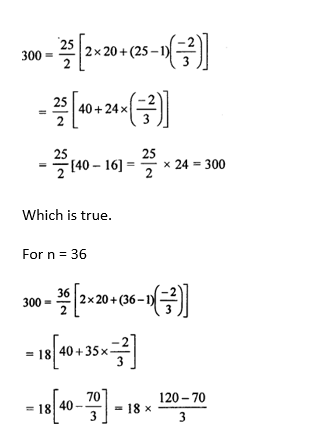= 300

Which is true.

Result is true for both the values of n

So both the numbers are correct.

Therefore, Sum of 11 terms is zero. (36-25 = 11)

Question 12: Find the sum of all odd numbers between 0 and 50.

Solution:

Odd numbers between 0 and 50 are 1, 3, 5, 7, 9, …, 49

Here, a = 1, d = 3 – 1 = 2, l = 49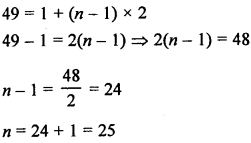Sum of odd numbers:

= n/2(a + l)

= 25/2 (l + 49)

= 25/2(50)

= 625

Question 13: Find the sum of all natural numbers between 200 and 400 which are divisible by 7.

Solution:

Natural numbers between 200 and 400 which are divisible by 7 are 203, 210, 217,……,399.

Here,AP is 203, 210, 217,……,399

First term = a = 203,

Common difference = d = 7 and

Last term = l = 399

We know that, l = a + (n-1)d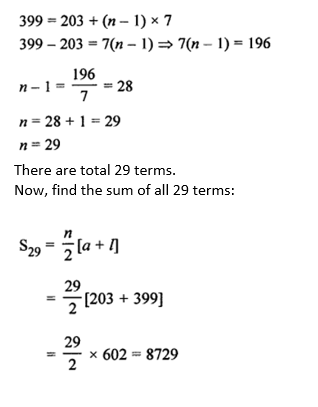Sum of all 29 terms is 8729.

Question 14: Find the sum of first forty positive integers divisible by 6.

Solution:

First forty positive integers which are divisible by 6 are

6, 12, 18, 24,…… to 40 terms

Here, a = 6, d = 12 – 6 = 6, and n = 40.

Sum of 40 terms: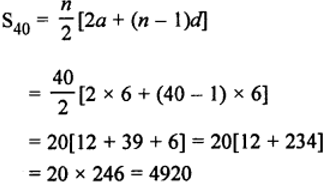Question 15: Find the sum of the first 15 multiples of 8.

Solution:

First 15 multiples of 8 are as given below:

8, 16, 24, 32,….. to 15 terms

Here, a = 8, d = 16 – 8 = 8, and n = 15

Sum of 15 terms: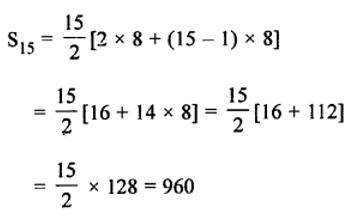Question 16: Find the sum of all multiples of 9 lying between 300 and 700.

Solution:

Multiples of 9 lying between 300 and 700 = 306, 315, 324, 333, …, 693

Here, a = 306, d = 9, and l = 693

We know that, l = a + (n-1)d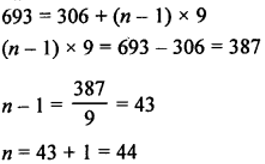There are 44 terms.

Find the sum of 44 terms: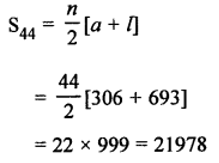Question 17: Find the sum of all three-digit natural numbers which are divisible by 13.

Solution:

3-digit natural numbers: 100, 101, 102,……., 999.

3-digit natural numbers divisible by 13:

104, 117, 130,………, 988

Which is an AP.

Here, a = 104, d = 13, l = 988

l = a + (n – 1) d

988 = 104 + (n-1)13

n = 69

There are 69 terms.

Find the sum of 69 terms:

Sn = n/2(a + l)

= 69/2(104 + 988)

= 37674

Question 18: Find the sum of first 100 even natural numbers which are divisible by 5.

Solution:

Even natural numbers: 2, 4, 6, 8, 10, …

Even natural numbers divisible by 5: 10, 20, 30, 40, … to 100 terms

Here, a = 10, d = 20 – 10 = 10, and n = 100

Sn = n/2(2a + (n-1)d)

= 100/2 [2 x 10 + (100-1)10]

= 50500

Question 19: Find the sum of the following.

(4 – 1/n) + (4-2/n) + (4– 3/n) + ….

Solution:

Given sum can be written as (4 + 4 + 4 + 4+….) – (1/n, 2/n, 3/n, …..)

Now, We have two series:

First series: = 4 + 4 + 4 + …… up to n terms

= 4n

Second series: 1/n, 2/n, 3/n, …..

Here, first term = a = 1/n

Common difference = d = (2/n) – (1/n) = (1/n)

Sum of n terms formula:

Sn = n/2[2a + (n – 1)d]

Sum of n terms of second series:

Sn = n/2 [2(1/n) + (n – 1)(1/n)]

= n/2 [(2/n) + 1 – (1/n)]

= (n + 1)/2

Hence,

Sum of n terms of the given series = Sum of n terms of first series – Sum of n terms of second series

= 4n – (n + 1)/2

= (8n – n – 1)/2

= 1/2 (7n – 1)

Question 20: In an AP, it is given that S5 + S7 = 167 and S10 = 235, then find the AP, where Sn denotes the sum of its first n terms.

Solution:

Let a be the first term and d be the common difference of the AP.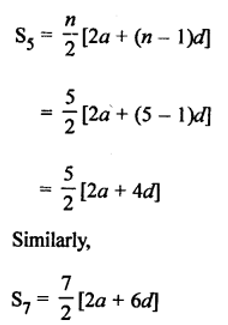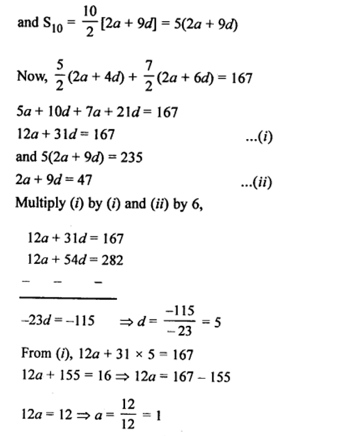Which implies: a = 1 and d = 5

Therefore, the AP is 1, 6, 11, 16,……

Question 21: In an AP, the first term is 2, the last term is 29 and the sum of all the terms is 155. Find the common difference.

Solution:

Let d be the common difference.

Given:

first term = a = 2

last term = l = 29

Sum of all the terms = Sn = 155

Sn = n/2[a + l]

155 = n/2[2 + 29]

n = 10

There are 10 terms in total.

Therefore, 29 is the 10th term of the AP.

Now, 29 = a + (10 – 1)d

29 = 2 + 9d

27 = 9d

d = 3

The common difference is 3.

Question 22: In an AP, the first term is -4, the last term is 29 and the sum of all its terms is 150. Find its common difference.

Solution:

Let d be the common difference.

Given:

first term = a = -4

last term = l = 29

Sum of all the terms = Sn = 150

Sn = n/2[a + l]

150 = n/2[-4 + 29]

n = 12

There are 12 terms in total.

Therefore, 29 is the 12th term of the AP.

Now, 29 = -4 + (12 – 1)d

29 = -4 + 11d

d = 3

The common difference is 3.

Question 23: The first and the last terms of an AP are 17 and 350 respectively. If the common difference is 9, how many terms are there and what is their sum?

Solution:

Let n be the total number of terms.

Given:

First term = a = 17

Last term = l = 350

Common difference = d = 9

l = a + (n-1)d

350 = 17 + (n-1)9

n = 38

Again,

Sn = n/2[a + l]

= 38/2[17 + 350]

= 6973

There are 38 terms in total and their sum is 6973.

Question 24: The first and the last terms of an AP are 5 and 45 respectively. If the sum of all its terms is 400, find the common difference and the number of terms.

Solution:

Let n be the total number of terms and d be the common difference.

Given:

first term = a = 5

last term = l = 45

Sum of all terms = Sn = 400

Sn = n/2[a + l]

400 = n/2[5 + 45]

n/2 = 400/50

n = 16

There are 16 terms in the AP.

Therefore, 45 is the 16th term of the AP.

45 = a + (16 – 1)d

45 = 5 + 15d

40 = 15d

15d = 40

d = 8/3

Common difference = d = 8/3

Common difference is 8/3 and the number of terms are 16.

Question 25: In an AP, the first term is 22, nth term is -11 and sum of first n terms is 66. Find n and hence find the common difference.

Solution:

Let n be the total number of terms and d be the common difference.

Given:

first term = a = 22

nth term = -11

Sum of all terms = Sn = 66

Sn = n/2[a + l]

66 = n/2[22 + (-11)]

n = 12

There are 12 terms in the AP.

Since nth term is -11, so

an = a + (n – 1)d

-11 = 22 + (12-1)d

d = -3

Therefore, Common difference is -3 and the number of terms are 12.

## R S Aggarwal Solutions for Class 10 Maths Chapter 5 Arithmetic Progression Exercise 5C

Class 10 Maths Chapter 5 Arithmetic Progression Exercise 5C is based on the following topic:

• Sum of n terms of an AP

Sum of n terms of AP formula: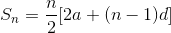First term = a

Common difference = d

Number of terms = n

R S Aggarwal Solutions for Class 10 will help students to practice more questions and master various concepts.